Have you heard off or ever tried Access Database DLookup function? Want to know about this Access Database DLookup function more? Then in that case you are at the correct platform. As, this tutorial is written with the main prospective of providing you with the complete information on MS Access Dlookup Function and mainly focus on how to use dlookup in Access.

### What’s This Access Dlookup Function Is?Microsoft Access application has a powerful data handling package with many strong functions to make data extraction like work in an easy way. From this vast collection of MS Access DLookup function, is the most workful one.

In MS Access application DLookup function is used to get value of particular field from specified set of records (a domain). You can use this DLookup function in Visual Basic for Applications (VBA) module, a query expression, a macro, or a calculated control on a form or report.

This function is best to retrieve value of particular field from a domain. Here domain can be a specified set of records from table, query or SQL expression. One can use this DLookup function to display value of fields which is not present in the underlying record set.

For example:

Suppose you have form on Order Details table. The form will display Quantity, OrderID, ProductID, UnitPrice, and Discount fields. However, in the product table “ProductName field” is present. In this case, you can use DLookup function in calculated control to display the ProductName on the same form.

### MS Access DLookup Function ExamplesDim newvar as variant

Newvar = DLookup(“[student name]”,”studentmaster”,”[rollno]=1001″)

From the above example extracts the data from field [student name] from the table studentmaster for roll number 1001.

Check what will happen if the DLookup function were given without any criteria.

Newvar = DLookup(“[student name]”,”studentmaster”)

Above code will return data in the field [student name] from the first record of the table studentmaster.

You can use variables in the criteria too. For instance, a form field can be used like<condition>

You may also like:

Learn 5 Different Ways To Create Forms In Microsoft Access Database …!

Newvar = DLookup(“[student name]”,”studentmaster”,”[rollno]=Form![rollno]”)

This will help in comparing the data in [student name] field from the table studentmaster with the one that is in the [rollno] field of the current form.

In order to specify textual criteria which is from a field on a form include the single quotes.

Nvar = DLookup(“[rollno]”,”studentmaster”,

“[student name]=’ ” & Forms![Marks]![student name] & ” ‘ “)

Overall, the moral of the story is Access DLookup is used as an expression in MS Access query or calculated field in form to retrieve foreign values. Well you can use this also in the Criteria field of the query builder.  That’s the reason why it is more efficient to make new query having fields of both tables and use it as base record set.

Access DLookup chooses the saved records of domain.  Other unsaved record is not included in the domain.

## Microsoft Access DLookup Function Syntax:DLookup( expr, domain [, criteria)

The DLookup function syntax has these arguments:

### Expr:

The Expression part identifies the field whose value you want to return. This can be a string expression to identify a field in table or query or it can be an expression which performs calculation on the field data.

In the DLookup Access Function Syntax’s expression part you can include name of field in a table, control or a form, constant or function. If this part includes any function then it can be either user-defined or built in but not SQL aggregate function or any other domain aggregate function.

### Domain

String expression which identifies the set of records constitutes the domain portion of the Access DLookup Function Syntax. It can be table name or query name for the query that don’t need any parameter.

### Criteria

String expression used to limit the range of data on which DLookup function is performed. Suppose, criteria is equivalent to WHERE clause in SQL expression, without using the word WHERE. If criteria is not mentioned then the DLookup function will be applied over the entire domain. Any field which is included in criteria must be a field in domain otherwise, the function will return a NULL value.

Have a look:

Learn All About Microsoft Access VBA Code – VBA Functions, Shortcuts And Much More…!

Access  database DLookup() function returns single value from a single field. If no record matches the criteria or if domain is empty DLookup() function will returns a Null value.  If more than one record matches the criteria, then in that case DLookup will return the first instance of that <fieldname>.

So, criteria should be defined carefully in order to get unique field from the DLookup function. If your criteria filed is text then keep that text in single quotation marks.

To calculate date or time values, enclose time or data value in number signs (#).

## How To Use DLookup() Function In Access?Here are the following circumstances explained to learn briefly about how to use Dlookup() Function in Access.

### #1. DLookup on Empty Data Record

If you apply the Access DLookup function over the table or query that does contain any records then it will show error message stating “Invalid Use of Null”. This happen because you are attempting to assign null value to the variable strX.

##### Code:

Dim strX As String

strX = DLookup(“HolidayDate”, “tblHolidays”)

Me.txtResult = strX

End Sub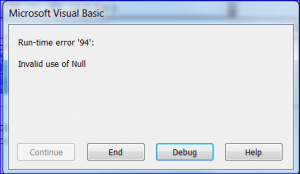In order to avoid this error message, you can make use of the IF statement to check whether there is record found before allotting result value to the strX.

##### Correct Code:

Private Sub Command0_Click()

Dim strX As String

If IsNull(DLookup(“HolidayDate”, “tblHolidays”)) Then

MsgBox “No Record Found”  ‘ or do something

Else

strX = DLookup(“HolidayDate”, “tblHolidays”)

Me.txtResult = strX

End If

End Sub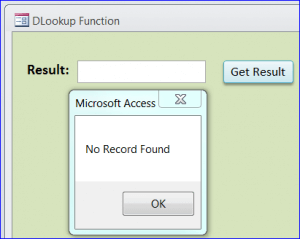### #2. DLookup with Textual Criteria

When you use sign of equal in the code “[UserLoginID] = ‘John’”, only the record having the entire User Name  = “John” will be appear in result of this criteria.

The result will displayed as “No Record Found” because there is no record with the user name “John”, but there are records for “John Smith” or “John Vang”.

##### Code:

Private Sub Command0_Click()

If IsNull(DLookup(“[User Name]”, “tblUser”, “[User Name] = ‘john'”)) Then

Me.txtResult = “No Record Found”

Else

Me.txtUserName = DLookup(“[User Name]”, “tblUser”, “[User Name] = ‘john'”)

End If

End Sub

### #3. DLookup without Criteria

Well it is the most simplest way to use DLookup() function ; i.e without specifying any criteria. In this case DLookup function will gives you the value of the first record in the CustomerName field from tbl_Customer table.

So, the output will be “Barney Rubble” because it is first record on tbl_Customer table as shown in the below figure.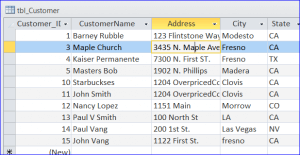##### Code:

Private Sub Command0_Click()

Dim strX As String

strX = DLookup(“CustomerName”, “tbl_customer”)

Me.txtResult = strX

End Sub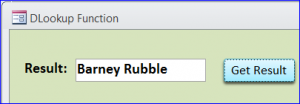### #4. DLookup with a Numeric Criteria that comes from the field on current Form

The code “[UserID] = Me.txtUserID” is used here as numeric criteria for DLookup in Access function.  This will show the output “John Vang” bacuse the UserID 3 is for the User Name “John Vang” as per the tblUser table shown above.

##### Code:

Private Sub cmdGetResult_Click()

Me.Refresh

If IsNull(Me.txtUserID) Then

Else

If IsNull(DLookup(“[User Name]”, “tblUser”, “[UserID] = ” & Me.txtUserID)) Then

Else

Me.txtUserName = DLookup(“[User Name]”, “tblUser”, “[UserID] = ” & Me.txtUserID)

End If

End If

End Sub

number user id from current form

DON’T MISS IT:

How To Create Pivot Table In MS Access And Resolve It’s Related Issue

### #5. DLookup with Textual Criteria that comes from a Field on Current Form

The code “[UserLoginID] = ‘” & Me.txtLoginID & “‘” is used as criteria for DLookup Access function.

Suppose, you want to get the user name for the entered UserLoginID on text box UserLoginID on this form then show it over the User Name text box .

It’s wrong if on the text box UserLoginID you enter jvang, the User Name should be “John Vang”. Here two IF Statements are used for this code to check whether the UserLoginID is entered or not and also to avoid error if User Name is left empty for the entered UserLoginID.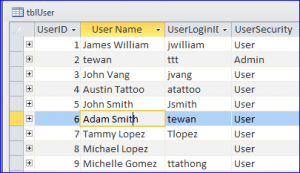##### Code:

Private Sub cmdGetResult_Click()

Me.Refresh

Else

If IsNull(DLookup(“[User Name]”, “tblUser”, “[UserLoginID] = ‘” & Me.txtLoginID & “‘”)) Then

MsgBox “No User Name for this UserLoginID”

Else

End If

End If

End Sub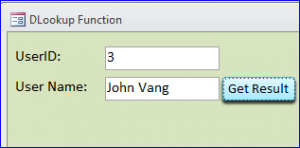### #6. DLookup Query with Date Criteria

DLookup MS Access function is also used to look information on query.  The code “[FinishDate] = #4/30/2014#” is been used as date criteria for the DLookup in Access. After applying the below code, the output will be “Task 5″ because the “Task 5” has the last date 4/30/2014 as the query “task completed” below.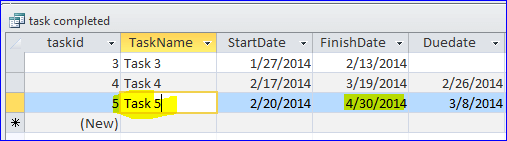##### Code:

Private Sub Command0_Click()

Me.txtResult = “No Record Found”

Else

End If

End Sub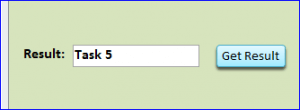### #7. DLookup with Numeric Criteria

In this section the code “[UserID] = 6” is used as numeric criteria for MS Access DLookup function.  This will show the output, the “Adam Smith” because UserID 6  is assigned for the User Name “Adam Smith.”##### Code:

Private Sub Command0_Click()

If IsNull(DLookup(“[User Name]”, “tblUser”, “[UserID] = 6”)) Then

Else

Me.txtUserName = DLookup(“[User Name]”, “tblUser”, “[UserID] = 6”)

End If

End Sub

### #8. DLookup with VBA Code

##### Example in VBA Code

You can also use the DLookup function with VBA code in Access. Here is the following example to learn how to use DLookup with VBA Code:

```Dim LDate As Date

LDate = DLookup("OrderDate", "Orders", "OrderID = 10248")```

In the above example, LDate is used as variable that will keep the OrderDate value of Orders table. From this Orders table, OrderID 10248 is used in the code.

### Wrap Up:

Hopefully all the aforementioned information about MS Access DLookup function seems helpful to you in easy accessing of this DLookup( ) function in Access Database from now onwards. The DLOOKUP function is quiet easy to use but after only when you understand how to work with it.  So, learn how to use DLOOKUP Access Function in multiple criteria and try them all. If you stuck into any further issue regarding DLookup Access function then ask in our FAQ section. You will surely get immediate response on your query.7 Ways To Use MS Access DLookup( ) Function That You Can’t Miss…!